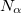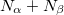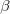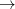Q-Chem 5.0 User’s Manual

The $molecule and$rem sections are required for all Q-Chem jobs, but depending on the details of the job a number of other input sections may be required. These are summarized briefly below, with references to more detailed descriptions to be found later in this manual.

By setting ECP = GEN, the user indicates that the effective core potentials (pseudopotentials, which replace explicit core electrons) to be used will be defined by the user. In that case, the $ecp section is used to specify these pseudopotentials. See Chapter 8 for further details. ## 3.5.4 User-Defined Exchange-Correlation Density Functionals ($xc_functional)

If the keyword EXCHANGE = GEN then a DFT calculation will be performed using a user-specified combination of exchange and correlation functional(s), as described in Chapter 4. Custom functionals of this sort can be constructed as any linear combination of exchange and/or correlation functionals that are supported by Q-Chem; see Section 4.4.4 for a list of supported functionals. The format for the $xc_functional input section is the following: $xc_functional
X  exchange_symbol  coefficient
X  exchange_symbol  coefficient
...
C  correlation_symbol  coefficient
C  correlation_symbol  coefficient
...
K  coefficient

If a user wants to change from the default values recommended by Grimme, then user-defined parameters can be specified using the $empirical_dispersion input section. See Section 4.4.7.2 for details. ## 3.5.6 Addition of External Point Charges ($external_charges)

If the $external_charges keyword is present, Q-Chem scans for a set of external charges to be incorporated into a calculation. The format is shown below and consists of Cartesian coordinates and the value of the point charge, with one charge per line. The charge is in atomic units and the coordinates are in ngstroms, unless bohrs are selected by setting the$rem keyword INPUT_BOHR to TRUE. The external charges are rotated with the molecule into the standard nuclear orientation.

Example 3.14  General format for incorporating a set of external charges.

$external_charges x-coord1 y-coord1 z-coord1 charge1 x-coord2 y-coord2 z-coord2 charge2 x-coord3 y-coord3 z-coord3 charge3$end


In addition, the user can request to add a charged cage around the molecule (for so-called “charge stabilization” calculations) using the keyword ADD_CHARGED_CAGE. See Section 6.7.6 for details.

## 3.5.7 Applying a Multipole Field ($multipole_field) A multipole field can be applied to the molecule under investigation by specifying the$multipole_field input section. Each line in this section consists of a single component of the applied field, in the following format.

Example 3.15  General format for imposing a multipole field.

$multipole_field field_component_1 value_1 field_component_2 value_2$end


Each field_component is stipulated using the Cartesian representation e.g., X, Y, and/or Z, (dipole field components); XX, XY, and/or YY (quadrupole field components); XXX, XXY, etc.. The value (magnitude) of each field component should be provided in atomic units.

## 3.5.8 User-Defined Occupied Guess Orbitals ($occupied and$swap_occupied_virtual)

It is sometimes useful for the occupied guess orbitals to be different from the lowest(or) orbitals. Q-Chem allows the occupied guess orbitals to be defined using the $occupied keyword. Using the$occupied input section, the user can choose which orbitals (by number) to occupy by specifying the-spin orbitals on the first line of the $occupied section and the-spin orbitals on the second line. For large molecules where only a few occupiedvirtual promotions are desired, it is simpler to use the$swap_occupied_virtual input section. Details can be found in Section 4.5.4.

## 3.5.12 Effective Fragment Potential Calculations ($efp_fragments and$efp_params)

These keywords are used to specify positions and parameters for effective fragments in EFP calculations. Details are provided in Section 11.5.

The $plots part of the input permits the evaluation of molecular orbitals, densities, electrostatic potentials, transition densities, electron attachment and detachment densities on a user-defined mesh of points. Q-Chem will print out the raw data, but can also format these data into the form of a “cube” file that is a standard input format for volumetric data that can be read various visualization programs. See Section 10.5 for details. ## 3.5.15 Intracules ($intracule)

Setting the $rem keyword INTRACULE = TRUE requests a molecular intracule calculation, in which case additional customization is possible using the$intracule input section. See Section 10.10.